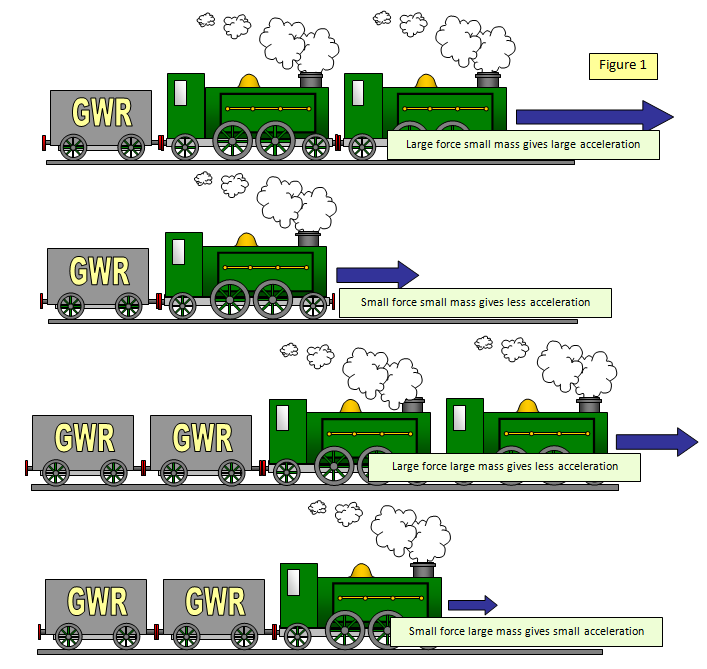# NEWTON'S SECOND LAW OF MOTION

Although no force is needed to keep things moving at a constant velocity Newton realised that you must have a force if you want to change the velocity of an object.

If an unbalanced force acts on an object then its velocity will change - it will either change direction, speed up or slow down, and that includes stopping.

Newton's second law explains how this change of velocity, or acceleration, is related to the mass of the body and the force applied.

If a 10 litre bus engine were fitted in a Mini - the acceleration would be impressive, but an 80 kg male sprinter would not accelerate off the starting blocks very quickly if his legs could only develop the same force as those of a grasshopper. However the grasshopper's legs give a great acceleration to a grasshopper!

The diagrams in Figure 1 show what would happen to different trains when different forces are applied to them.Experiments and theory show that:
(a) the greater the force for a given mass the greater the acceleration
(b) the smaller the mass for a given force the greater the acceleration
These results can be summarised as follows:

An unbalanced force produces an acceleration

Newton stated these results in his second law of motion which is:

Force = mass x acceleration
F= ma

The force (F) must be in Newtons, the mass (m) in kilograms and the acceleration (a) in metres per second per second.

This formula can be used to define the unit of force – the NEWTON.

A force of one Newton will give a mass of one kilogram an acceleration of 1 m/s2

Examples
1. A trolley with a mass of 15 kg is accelerated at 3 m/s2. What force is need to do this?
F = ma = 15 x 3 = 45 N

2. What is the acceleration of a 4 kg box if an unbalanced force of 12 N acts on it?
F = ma   a = F/m = 12/4 = 3 m/s2

3. A force of 20N is used to accelerate a 250 g mass. What is its acceleration?
F = ma   a = 20/0.25 = 80 m/s2

4. When an object of unknown mass has an unbalanced force of 200N acting on it it accelerates at 2 m/s2. What is its mass?
F = ma    m = F/a = 200/2 = 100 kg
(A useful way of measuring mass in weightless conditions!)### schoolphysics Newton's trains animation

To see an animation of the effects of Newton's second law on the motion of trains click on the animation link.### schoolphysics Newton's trains animation

To see an animation of the effects of Newton's second law on the motion of trains that will also work on tablets click on the second animation link.

A VERSION IN WORD IS AVAILABLE ON THE SCHOOLPHYSICS CD